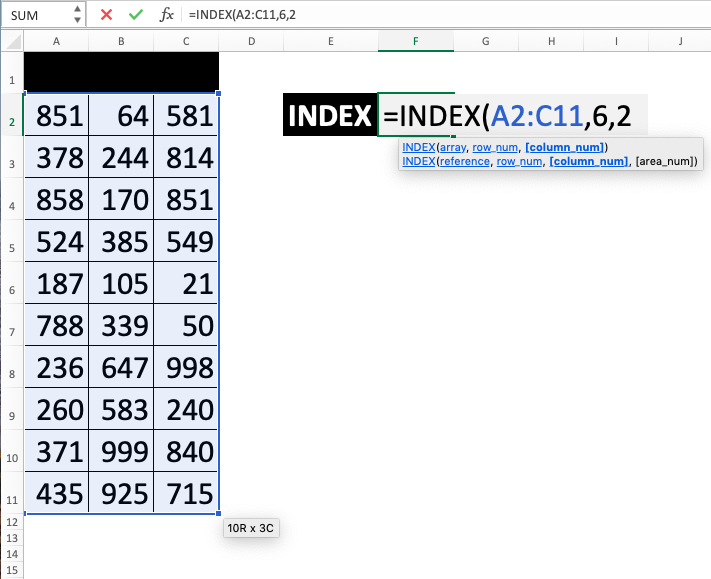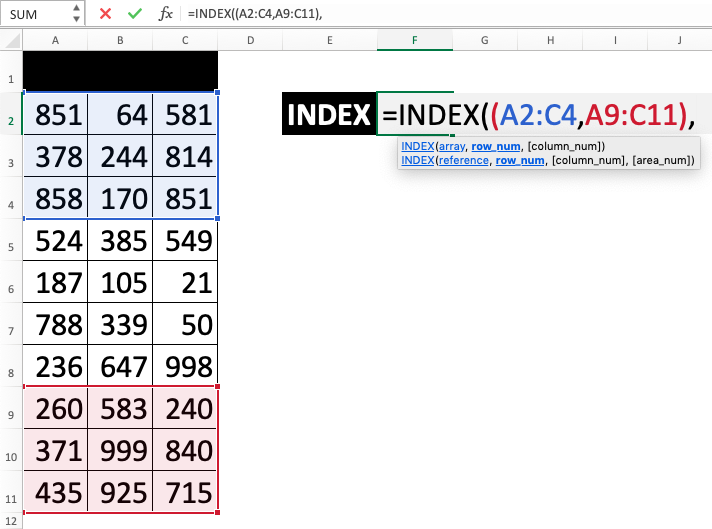INDEX Function in Excel - Compute Expert

# INDEX Function in Excel

In this tutorial, you will learn about how to use INDEX formula in excel. INDEX function in excel is something that can be used to find data in a cell range based on our row and column positions input. INDEX formula in excel has two kinds of input writing that can be utilized although you probably will mostly only use one of them.

## Why We Need to Learn About INDEX Formula in Excel?

In the process of searching and finding data in a spreadsheet, sometimes we need to pull data after we have known the row and column position of the data. This is needed so we can separate it from the data group column and row that we have and it can be processed further on different parts in the spreadsheet software.

For a case example in work and business, imagine if we have a table consists of sales branches performance in the company with many indicators for the performance shown in a spreadsheet file. Maybe we want to process the indicator in another place as needed. If the data pulling process is done from a group of columns and rows with a small number of data, then it will be not hard to do it manually. But if there is a large number, it is better to do it automatically so it will not take too much time for the separation process.

In this spreadsheet tool, there is INDEX function in excel to help the process. In the previous case, we should already know about the row and column positions and all the data which want to be pulled should be in orderly positions. Thus, this formula will be very helpful in separating all of them (by doing formula copy after the initial one is written). If you often need to do a separation process like that with the data position that wants to be separated is already known, then INDEX formula in excel can be very valuable to be understood.

Besides that, INDEX function in excel usage is very useful to be known if it is combined with MATCH usage to find data based on certain indicators. The usage objective of those formulas combination is pretty similar to VLOOKUP and HLOOKUP but the INDEX formula in excel with MATCH combination should be easier and more flexible to write if you have understood how to use the two formulas combination.

## What is INDEX Function in Excel?

INDEX function in excel is a function with the usage objective to pull data from a cell range by telling the position of the data in the cell range. This function is often used with MATCH so the finding process done by the function can be practiced in a more dynamic way

Below is the brief explanation about the inputs in these formulas. There are two kinds of inputs that can be used with the first kind below is the most often used with MATCH.

=INDEX(array, row_num, column_num)

Note:
• array = the cell range where you want to take your data
• row_num = the row position in your cell range where the data is
• column_num = the row position in your cell range where the data is

=INDEX(reference, row_num, column_num, area_num)

Note:
• reference = a group of cell ranges where you want to take your data (more than one)
• row_num = the row position in your selected cell range where the data is
• column_num = the row position in your selected cell range where the data is
• area_num = the position of your selected cell range in your first input to find the data

## How to Use INDEX Formula in Excel?

The following will explain how to use INDEX function in excel. INDEX formula in excel has two kinds of input groups that can be used. The first one is the inputs of the cell range where the finding process will be done and the positions of row and column of the data. The second input group is the inputs of more than one cell ranges for reference, the positions of row and column of the data in the chosen cell range, and the position of chosen cell range on the reference input as the searching place. In the next part, we will explain about the writing method of those two input groups in this INDEX function in excel.

## Using INDEX Function in Excel (First)

1. Type equal sign ( = ) in the cell where you want to put the result2. Type INDEX (can be with large and small letters) and open bracket sign after =3. Drag cursor from the most top-left coordinate until the most bottom right for the cell range where the finding process of the data will be held and then type comma sign ( , )4. Type number of row position or cell coordinate where that number is then type comma sign5. Type number of column position or cell coordinate where that number is6. Type close bracket sign7. Press Enter
8. The process is done!## Using INDEX Function in Excel (Second)

1. Type equal sign ( = ) in the cell where you want to put the result2. Type INDEX (can be with large and small letters) and open bracket sign after =3. Input reference of all cell ranges by dragging the cursor from the most top-left coordinate until the most bottom right for all the cell ranges where the finding process can be held. Separate all of the cell ranges with comma sign ( , )4. Type close bracket sign then type comma sign5. Type number of row position in later chosen cell range or cell coordinate where that number is then type comma sign6. Type number of column position in later chosen cell range or cell coordinate where that number is7. Type number that represents which cell range from the reference input will be used or the cell coordinate where that number is. That number is the position of cell range input that is chosen from your bracket in the previous reference input8. Type close bracket sign9. Press Enter
10. The process is done!## Exercise

After you have learned the usage steps of INDEX formula in excel in the tutorial, you can practice your understanding to be more adept by working on the exercise below!

### Questions

Use INDEX formula in excel to answer the following three questions!
1. How many is the sales of product F?
2. How many products in region II are in the sending process?
3. How many stocks does product J have? Use second method of INDEX formula in excel to answer it!

• The result of INDEX formula in excel is cell(s) coordinate not the data directly. Because of that, probably it can be useful if it is combined with another formula which has that kind of input
• If the row position input is given 0, then the result of INDEX function in excel is one column coordinate and if the input of the column position is given 0, then the result of this INDEX formula in excel is one row coordinate. This can be useful if the result is combined with another formula which can use the coordinate of one row/column
• Learn MATCH formula in another part of Compute Expert tutorial blog to optimize INDEX function in excel usage!

Get updated excel info from Compute Expert by registering your email. It's free!